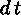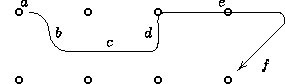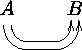## 2.8 More bending arrows

Finally, arrows can bend around entries: just insert ```, i.e., a backquote and a direction d followed by a target t, for each "turn" that starts out in the d-direction and ends in a quarter turn towards the target t. This is different from the curving described earlier in that all turns consist of a straight part ending in a turn which is a multiple of 18 circle segments, and each segment allows separate labels.

The possible directions are those of section 1.6 and 2.6, and the possible targets include all those discussed above. Actually the direction is only required for the first in a series of turns since the final direction of one turn is the obvious choice for the following turn. Furthermore, turns can be changed from the default by adding either `^`d for anticlockwise turn to d or `_`d for clockwise turn to d, where d is the "exit direction" of the turn.

Finally, the turns will have radius 10pt by default, but this can be changed to any dimension R from a particular turn and onwards by inserting `/`R immediately after the `"` of the turn. Here is an example involving all of these features:

```\xymatrix{
{\circ} \ar `r[d]   ^a
`[rr]     ^b
`/4pt[rr] ^c
`[rrr]    ^d
`_dl[drrr]^e
[drrr]^f
& {\circ} & {\circ} & {\circ} \\
{\circ} & {\circ} & {\circ} & {\circ} }```

typesetsThe example illustrates the following points:

• If the segment can not be made as short as required then it will point `past" the target. This is useful for "going around" entries.
• There is one ``` per turn thus each target appears as many times as there are turns towards it, except the last target that appears one more time namely both with ``` for each turn towards it and once as an "ordinary" target to set the final stretch.
• The sizes of the intermediate targets are ignored.

#### Exercise 13.

Typeset.

© Kristoffer H. Rose <krisrose@brics.dk> December 19, 1996 / LaTeX2HTMLv96.1-h